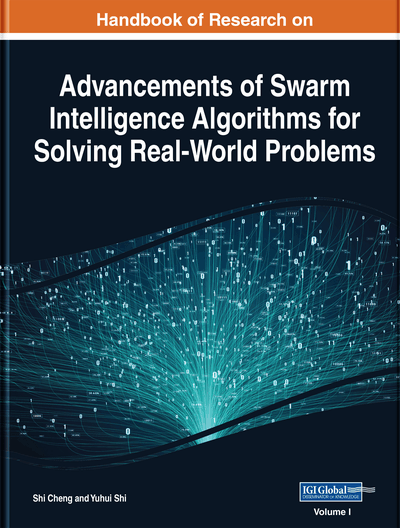# Analysis of the Dynamic Characteristics of the Firefly Algorithm

Takuya Shindo
DOI: 10.4018/978-1-7998-3222-5.ch006
OnDemand:
(Individual Chapters)
Available
\$29.50
No Current Special Offers

## Abstract

The firefly algorithm is a meta-heuristic algorithm, the fundamental principle of which mimics the characteristics associated with the blinking of natural fireflies. This chapter presents a rigorous analysis of the dynamics of the firefly algorithm, which the authors performed by applying a deterministic system that removes the stochastic factors from the state update equation. Depending on its parameters, the individual deterministic firefly algorithm exhibits chaotic behavior. This prompted us to investigate the relationship between the behavior of the algorithm and its parameters as well as the extent to which the chaotic behavior influences the searching ability of the algorithm.
Chapter Preview
Top

## Introduction

Under the given constraints, the Optimization Problem is to find a solution that a certain objective function gives the maximum value or the minimum value. The optimization problem has been studied in various fields such as engineering, economics, and et al.

In recent years, many researchers pay attention to swarm intelligence as the application of the optimization problem solver. The swarm intelligence algorithm is between agents to emerge the behavior by local interaction (Krishnanand and Ghose, 2008). For example, some methods are based on the behavior of ants colony, slime mold colony, fish flock, and so on, (Dorigo, Maniezzo and Colorni, 1996)(Zengin and Tuncel, 2010).

A number of meta-heuristic algorithms with diverse applications have been proposed. These algorithms are continuously being improved and studies are also underway to enhance the solution search performance of the algorithm. When considering such performance improvements, an analysis of the characteristics of the algorithm is very important. In general, the theoretical analysis of meta-heuristic algorithms is highly challenging because most of these algorithms contain stochastic factors, (Clerc & Kennedy, 2002; Koguma & Aiyoshi, 2010). Therefore, stochastic analysis of the operation of meta-heuristic algorithms is considered very difficult. Therefore, to analyze the dynamics of the firefly algorithm, the authors proposed a deterministic firefly algorithm.

The firefly algorithm (FA) is one of these meta-heuristic algorithms, (Yang, 2010; Yang, Ed. 2013). The FA was developed by Xin-She Yang et al. and is based on the characteristics of the blinking of a natural firefly. Many performance improvement methods and application fields have already been studied for the FA (Cai, Niu, & Yang, 2018; Alor, Mota, Olmos, & Rodas, 2019; Wang et al., 2018). The original FA expresses the dynamics of the firefly by a simple update equation; however, apart from this, the behavior of the FA is very complex.

The conventional FA is based on the following three rules, (Yang, 2010; Yang, Ed. 2013):

• 1.

Fireflies are unisex creatures such that one firefly will be attracted to other fireflies regardless of their sex.

• 2.

The attractiveness is of a firefly proportional to its brightness, with both of these properties decreasing as the distance between individual fireflies increases. Thus, for any two flashing fireflies, the less bright one would be expected to move towards the brighter one. In the absence of any firefly brighter than itself, it will move randomly.

• 3.

The brightness of a firefly is determined by the landscape of the objective function.

In the work presented in this chapter, the stochastic factors are removed from the update equation. Furthermore, the authors investigate and confirm the effect of the solution search performance of the FA when it exhibits chaotic behavior (Yang, 2013).

Top

## Firefly Algorithm

The dynamics of the FA are described by the following equation.

,(1) wheredenotes the position of the i-th firefly on the t-th iteration, β denotes the attractiveness of the i-th firefly as perceived by the j-th fireflies, αt represents the variation of the randomness with t anddenotes a random number vector on the t-th iteration.

## Complete Chapter List

Search this Book:
Reset